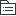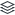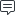# 游戏发行运营流程梳理

48
|
19

## 相关模板推荐

•## 发行运营流程梳理 —— 作品大纲

•产品立项
•1.从市场角度评估产品机会 2.对标竞品，做出差异化优势 3.微创新，没有足够把握避免做革命性创新
• 市场机会评估
• 立项初衷、产品定位
• 产品亮点挖掘
• IP、画风、世界观
• 玩法特色
• 对比竞品的差异化
• 市场定位
• 目标用户人群
• 用户画像分析
• 渠道定位
• 产品评测
• 商业化设计
• 本地化设计
• 付费思路
• 高arpu，低付费率
• 低arpu，高付费率
• 对不同层次玩家的友好度
• 美术、底层优化
• CP评估
• CP技术实力
• 过往产品情况
• CP运维实力
•发行立项
•根据产品特性制定市场策略
• 用户画像
• 性别
• 年龄
• 消费习惯
• 爱好话题
• 游戏时长定位
• 用户分层：核心、次核心、泛用户
• 包装策略（素材策略）
• 产品IP、题材、画风定位
• 产品形象
• 包装文案
• 广告形式、广告内容
• 针对不同人群的包装策略
• 视觉策略
• 色调
• 3D or 2D
• 动作特写
• 主题风格
• 流量策略
• 流量构成
• 渠道、买量占比
• 根据数据预估模型推算目标量级
• 渠道策略
• 重点发行渠道
• 资源位策略
• 苹果推荐策略
• 预约方案
• 买量策略
• 买量用户群体
• 买量节奏
• 社区运营方案
• 市场预算评估
•产品打磨（秘密测试）
•1.测试买量数据，要求数据达标才能进行下一步市场计划 2.深入介入到产品商业化工作，从市场角度提出优化意见
• 版本验收
• 本地化进度
• 商业化进度
• 版本内容跟进
• 技术支持
• 运营后台对接
• 数据上报打点排查
• 数据后台接入
• 客户端性能检验
• 反外挂测试
• 测试排期
• 测试目的
• 吸量测试
• 留存测试
• 付费测试
• 测试人群
• 买量测试
• Q群测试
• 测试量级
• 时间节点
• 关注的数据指标及目标预期
• 服务器策略
• 开服节奏
• 单服生态量级
• 复盘分析
• 数据复盘
• 玩家意见收集
• 产品优化方案
• 迭代的版本内容
• 循环测试直到数据达标
•市场预热
•1.必须在产品数据达标后再启动市场计划，避免市场先行 2.市场、商务、运营需要聚拢KPI指标，共同为关键指标负责
• 市场发行目标
• 预约
• 下载
• 新增
• 市场执行方案
• 视觉包装方案
• 线上、线下宣传预约策略
• 漫展
• 社区曝光
• PR稿
• 渠道策略确认
• 社群维护方案
• 市场费用落实
• 跟踪确认数据情况
• 预约数
• 下载数
• 评论维护
• 新增转化漏斗
• 官网搭建
• 落地页
• 预约站
• 主页
•渠道测试
•1.维护渠道关系 2.按照排期节点准时上线 3.测试渠道数据
• 测试排期
• 删档不付费
• 删档付费
• 首发
• 渠道策略
• 测试的渠道范围
• 商务争取渠道资源位置
• 代金券、渠道活动等方案确定
• 预约曝光资源
• SDK接入
• 渠道执行工作
• 渠道物料准备
• 玩法介绍文稿
• 激活码
• 宣传素材
• 传包、提审
• 社区运营
• Q群建设
• 评论维护
• 复盘分析
• 数据复盘
• 调优规划
• 工作踩坑总结
•首发上线
•执行工作落实到位
• 版本验收
• 游戏内容
• 运营活动
• SDK接入
• 打点排查
• 数据后台验收
• 运营工具后台验收
• 客户端性能检验
• 封包确认
• 运营活动
• 活动目的
• 活动类型
• 活动力度
• 活动节奏
• 渠道工作筹备
• 节点排期、上线资源
• 传包、提审
• 代金券
• 渠道活动
• 社区文稿
• 服务器策略
• 开服节奏
• 单服生态导量人数
• 服务器压力测试
• 数据备份、清档
• 社区运营
• 评论维护
• 建立玩家群
• 其他内容
• 游戏公告
• 邮件模板
• 激活码筹备
•长线运营
•1.版本调优 2.通过活动调节生态、流水 3.掌握长线运营的核心节奏，避免朝三暮四 4.定期复盘
• KPI目标制定
• 新增
• 留存
• 流水
• 数据收集
• 数据日报、周报、月报
• 新增转化
• 预约转化、素材转化
• 下载、激活、更新、注册、登陆
• 持续的素材测试
• 产品数据分析
• 生态现状
• 版本效果
• 活动效果
• 产耗情况
• 关键节点流失
• 版本规划
• 版本节奏、内容、验收
• 关注产品数据
• 关注市场热点
• 配合版本的渠道资源、市场策略
• 版本效果复盘
• 运营活动
• 活动投放节奏
• 迭代活动类型
• 活动效果复盘
• 联运外放
• 联运合作对接